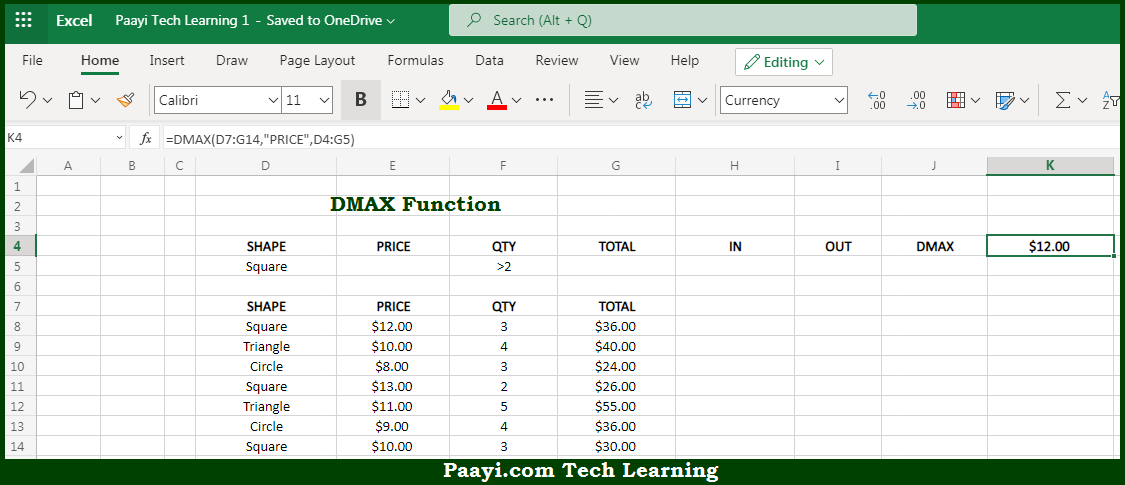# Learn How to Use Microsoft Excel DMAX Function

Written by | 0 Comments | 619 Views

In this article, you will learn how to use the Microsoft Excel DMAX function and its prime function in Microsoft Excel. You will also get to know the Microsoft Excel DMAX function return value and syntax with the help of some examples.

Microsoft Excel DMAX Function

The main purpose of the Microsoft Excel DMAX function is to get the maximum value from the matching records in the database. That implies, with the help of the DMAX function you can able to return the maximum value in a field, from a set of records that match criteria. You can use the DMIN function to get the minimum value. So, with the help of the DMAX function, you can able to get the maximum value from the matching records in the database.

Return Value of DMAX Function

The return value will be the maximum value in the given field.

Syntax of DMAX Function

=DMAX(database, field, criteria)

Where the arguments:

• database: This is the database range including headers.
• field: This is a field name or index to count optional.
• criteria: This is the criteria range including headers.

## How to Use Microsoft Excel DMAX Function?So we know that Microsoft Excel DMAX function you can able to get the maximum value from the matching records in the database. That implies, with the help of the DMAX function you can able to return the maximum value in a field, from a set of records that match criteria. You can use the DMIN function to get the minimum value. So, with the help of the DMAX function, you can able to get the maximum value from the matching records in the database.